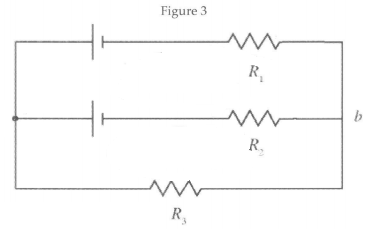# Problem: In Figure 3, consider the circuit sketched. The two batteries have negligible internal resistance and emf's  ε1 = 28.0 V and ε2 = 42.0 V. The three resistors have resistance  R1 = 2.00 Ω, R2 = 5.00 Ω, R3 = 1.00 Ω. Calculate the potential difference Va - Vb between points a and b.

###### FREE Expert Solution
88% (241 ratings)
###### Problem Details

In Figure 3, consider the circuit sketched. The two batteries have negligible internal resistance and emf's  ε1 = 28.0 V and ε2 = 42.0 V. The three resistors have resistance  R1 = 2.00 Ω, R2 = 5.00 Ω, R3 = 1.00 Ω. Calculate the potential difference Va - Vb between points a and b.Frequently Asked Questions

What scientific concept do you need to know in order to solve this problem?

Our tutors have indicated that to solve this problem you will need to apply the Kirchhoff's Loop Rule concept. You can view video lessons to learn Kirchhoff's Loop Rule. Or if you need more Kirchhoff's Loop Rule practice, you can also practice Kirchhoff's Loop Rule practice problems.

How long does this problem take to solve?

Our expert Physics tutor, Douglas took 17 minutes and 34 seconds to solve this problem. You can follow their steps in the video explanation above.

What professor is this problem relevant for?

Based on our data, we think this problem is relevant for Professor Ford's class at TAMU.